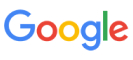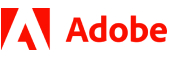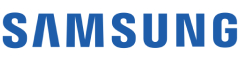New update is available. Click here to update.
Topics

# Colorful Knapsack

Hard0/120
Average time to solve is 45m+18 more companies

## Problem statement

You are given 'N' stones labeled from 1 to 'N'. The 'i-th' stone has the weight W[i]. There are 'M' colors labeled by integers from 1 to 'M'. The 'i-th' stone has the color C[i] which is an integer between 1 to 'M', both inclusive.

You have been required to fill a Knapsack with these stones. The Knapsack can hold a total weight of 'X'.

You are required to select exactly 'M' stones; one of each color. The sum of the weights of the stones must not exceed 'X'. Since you paid a premium for a Knapsack with capacity 'X', you are required to fill the Knapsack as much as possible.

Write a program to calculate the best way to fill the Knapsack - that is, the unused capacity should be minimized.

Detailed explanation ( Input/output format, Notes, Images )
Constraints :
``````1 <= M <= N <= 100
1 <= X <= 10000
1 <= W[i] <= 100
1 <= C[i] <= M

Time Limit: 1 sec
``````
Sample Input 1:
``````3 3 5
1 1 1
1 2 3
``````
Sample Output 1:
``````2
``````
Explanation of Sample Input 1:
``````We have three stones of each color and hence, we have to select it and in turn, we get a total weight equal to 1 + 1 + 1 = 3. So the minimum unused capacity is 5 - 3 = 2.
``````
Sample Input 2:
``````8 3 13
2 3 4 2 4 5 2 3
1 1 1 2 2 2 3 3
``````
Sample Output 2:
``````1
``````
Explanation of Sample Input 2:
``````We can choose the stone with:
1. Colour as 1 and with Weight 4,
2. Colour as 2 and with Weight 5, and
3. Colour as 3 and with Weight 3

So we a total weight 4 + 5 + 3 = 12. Hence the minimum unused capacity is 13 - 12 = 1.

We cannot get weight more than 12 with any other combination.
``````Console